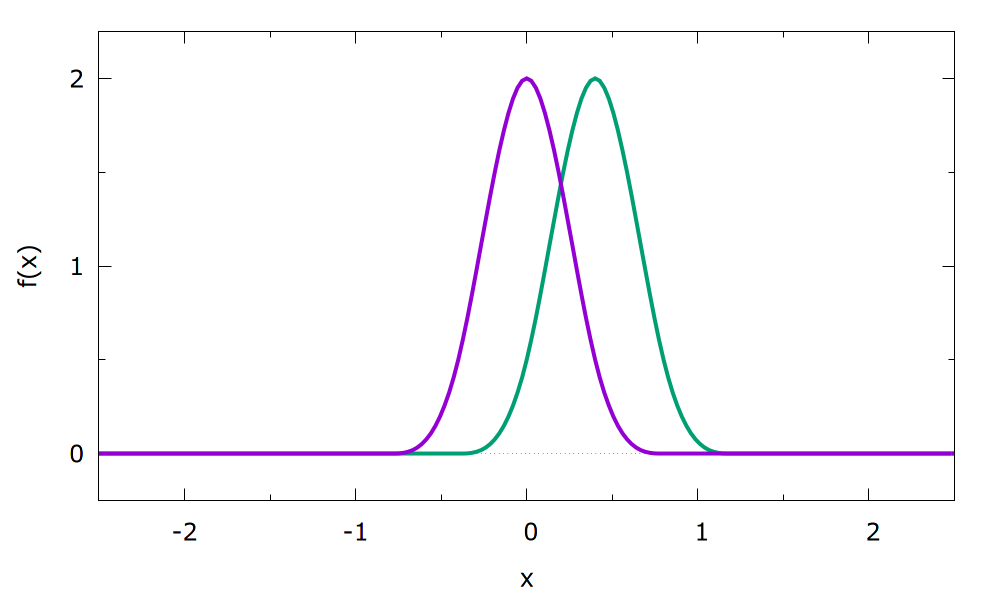BF_CUBIC_B_SPLINES
 This is part of the ves module It is only available if you configure PLUMED with ./configure –enable-modules=ves . Furthermore, this feature is still being developed so take care when using it and report any problems on the mailing list.

Cubic B spline basis functions.

Attention
These basis functions do not form orthogonal bases. We recommend using wavelets (BF_WAVELETS) instead that do for orthogonal bases.

A basis using cubic B spline functions according to . See  for full details.

The mathematical expression of the individual splines is given by

\begin{align*} h\left(x\right) = \begin{cases} \left(2 - \lvert x \rvert\right)^3, & 1 \leq \lvert x \rvert \leq 2\\ 4 - 6\lvert x \rvert^2 + 3 \lvert x \rvert^3,\qquad & \lvert x \rvert \leq 1\\ 0, & \text{elsewhere}. \end{cases} \end{align*}

The full basis consists of equidistant splines at positions $$\mu_i$$ which are optimized in their height:

\begin{align*} f_i\left(x\right) = h\left(\frac{x-\mu_i}{\sigma}\right) \end{align*}

Note that the distance between individual splines cannot be chosen freely but is equal to the width: $$\mu_{i+1} = \mu_{i} + \sigma$$.

The ORDER keyword of the basis set determines the number of equally sized sub-intervalls to be used. On the borders of each of these sub-intervalls the mean $$\mu_i$$ of a spline function is placed.

The total number of basis functions is $$\text{ORDER}+4$$ as the constant $$f_{0}(x)=1$$, as well as the two splines with means just outside the interval are also included.

As an example two adjacent basis functions can be seen below. The full basis consists of shifted splines in the full specified interval.When the splines are used for a periodic CV (with the PERIODIC keyword), the sub-intervals are chosen in the same way, but only $$\text{ORDER}+1$$ functions are required to fill it (the ones at the boundary coincide and the ones outside can be omitted).

To avoid 'blind' optimization of the basis functions outside the currently sampled area, it is often beneficial to use the OPTIMIZATION_THRESHOLD keyword of the VES_LINEAR_EXPANSION (set it to a small value, e.g. 1e-6)

Examples
The bias is expanded with cubic B splines in the intervall from 0.0 to 10.0 specifying an order of 20. This results in 24 basis functions.
Click on the labels of the actions for more information on what each action computesbf: BF_CUBIC_B_SPLINES MINIMUMcompulsory keyword
The minimum of the interval on which the basis functions are defined. =0.0 MAXIMUMcompulsory keyword
The maximum of the interval on which the basis functions are defined. =10.0 ORDERcompulsory keyword
The order of the basis function expansion. =20

Glossary of keywords and components
Compulsory keywords
 ORDER The order of the basis function expansion. MINIMUM The minimum of the interval on which the basis functions are defined. MAXIMUM The maximum of the interval on which the basis functions are defined.
Options
 DEBUG_INFO ( default=off ) Print out more detailed information about the basis set. Useful for debugging. PERIODIC ( default=off ) Use periodic version of basis set. NORMALIZATION the normalization factor that is used to normalize the basis functions by dividing the values. By default it is 2.